Question

True False O O 1. RETURN - LOSS / ORIGINAL COST O O O O O...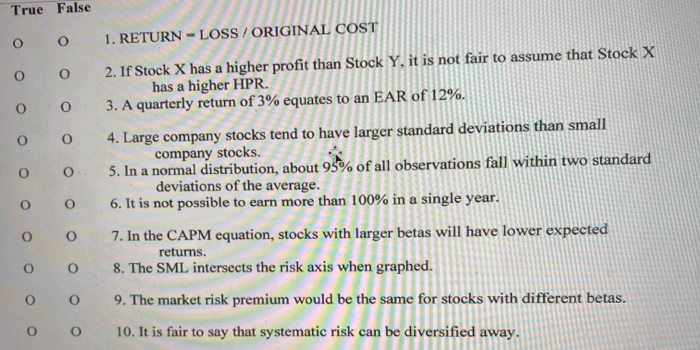True False O O 1. RETURN - LOSS / ORIGINAL COST O O O O O 0 2. If Stock X has a higher profit than Stock Y, it is not fair to assume that Stock X has a higher HPR. 3. A quarterly return of 3% equates to an EAR of 12%. 4. Large company stocks tend to have larger standard deviations than small company stocks. 5. In a normal distribution, about 95% of all observations fall within two standard deviations of the average. 6. It is not possible to earn more than 100% in a single year. O 0 O 0 O O O 0 7. In the CAPM equation, stocks with larger betas will have lower expected returns. 8. The SML intersects the risk axis when graphed. 9. The market risk premium would be the same for stocks with different betas. O O O O 10. It is fair to say that systematic risk can be diversified away.

1.
True

2.
True

3.
False, (1+3%)^4-1=12.5509%

4.
False, lower standard deviations

5.
True

6.
False, it is possible

7.
False, higher expected returns

8.
False, intercepts the return axis only

9.
True

10.
False, cannot be diversified away

Earn Coins

Coins can be redeemed for fabulous gifts.

Similar Homework Help Questions
• Which of the following is TRUE about RISK & RETURN? O A. Ceteris paribus, higher standard...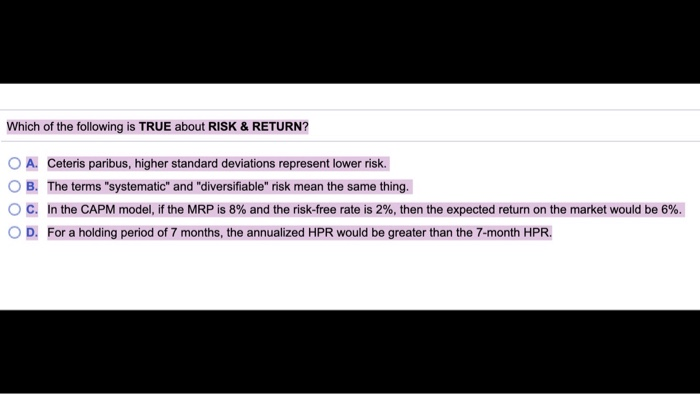Which of the following is TRUE about RISK & RETURN? O A. Ceteris paribus, higher standard deviations represent lower risk. O B. The terms "systematic" and "diversifiable" risk mean the same thing. O C. In the CAPM model, if the MRP is 8% and the risk-free rate is 2%, then the expected return on the market would be 6%. OD. For a holding period of 7 months, the annualized HPR would be greater than the 7-month HPR.

• 10-15 true or false for each statement 0 0 10. In the CAPM, stocks with larger...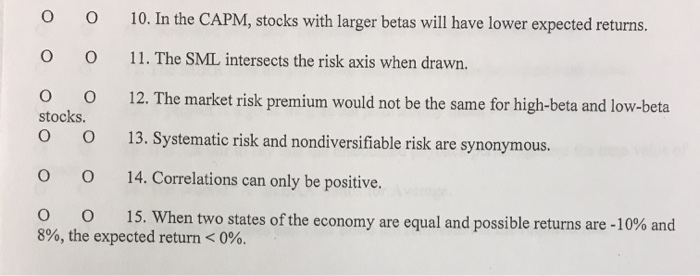10-15 true or false for each statement 0 0 10. In the CAPM, stocks with larger betas will have lower expected returns. 0 0 11. The SML intersects the risk axis when drawn. 12. The market risk premium would not be the same for high-beta and low-beta 0 0 stocks. 0 0 13. Systematic risk and nondiversifiable risk are synonymous. 0 0 14. Correlations can only be positive. 0 0 15. When two states of the economy are equal and...

• Suppose that the average excess return on stocks is 12.00% and that the risk-free interest rate...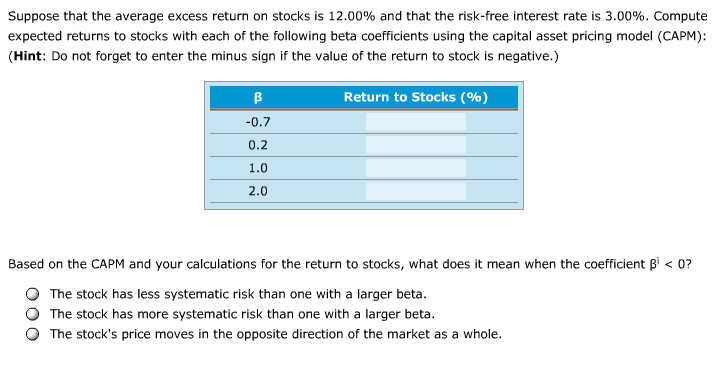Suppose that the average excess return on stocks is 12.00% and that the risk-free interest rate is 3.00%. Compute expected returns to stocks with each of the following beta coefficients using the capital asset pricing model (CAPM): Hint: Do not forget to enter the minus sign if the value of the return to stock is negative.) Return to Stocks (%) 0.7 0.2 1.0 2.0 Based on the CAPM and your calculations for the return to stocks, what does it mean...

• PLEASE EXPLAIN WHY ANSWER IS TRUE OR FALSE: "Risk aversion" implies that investors require higher expected...

PLEASE EXPLAIN WHY ANSWER IS TRUE OR FALSE: "Risk aversion" implies that investors require higher expected returns on riskier than on less risky securities. a.   True                                                    b.   False When adding a randomly chosen new stock to an existing portfolio, the higher (or more positive) the degree of correlation between the new stock and stocks already in the portfolio, the less the additional stock will reduce the portfolio's risk. a.   True b.   False An individual stock's diversifiable risk, which is measured...

• EVALUATING RISK AND RETURN Stock X has a 9.5% expected return, a beta coefficient of 0.8,...

EVALUATING RISK AND RETURN Stock X has a 9.5% expected return, a beta coefficient of 0.8, and a 35% standard deviation of expected returns. Stock Y has a 12.5% expected return, a beta coefficient of 1.2, and a 20.0% standard deviation. The risk-free rate is 6%, and the market risk premium is 5%. Calculate each stock's coefficient of variation. Round your answers to two decimal places. Do not round intermediate calculations. CVx = CVy = Which stock is riskier for...

• EVALUATING RISK AND RETURN Stock X has a 9.5% expected return, a beta coefficient of 0.8,...

EVALUATING RISK AND RETURN Stock X has a 9.5% expected return, a beta coefficient of 0.8, and a 40% standard deviation of expected returns. Stock Y has a 12.5% expected return, a beta coefficient of 1.2, and a 20.0% standard deviation. The risk-free rate is 6%, and the market risk premium is 5%. a. Calculate each stock's coefficient of variation. Round your answers to two decimal places. Do not round intermediate calculations. CVx = ________ CVy = ________ b. Which...

• The following graph plots the current security market line (SML) and indicates the return that investors...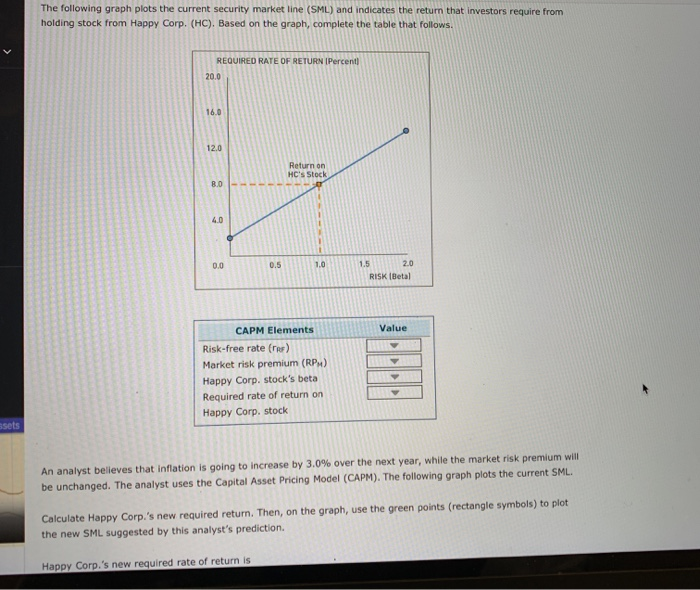The following graph plots the current security market line (SML) and indicates the return that investors require from holding stock from Happy Corp. (HC). Based on the graph, complete the table that follows. REQUIRED RATE OF RETURN IPercent) 20.0 16.0 12.0 Return on HC's Stock 8.0 4.0 2.0 1,0 1.5 0.5 0.0 RISK 1Betal Value CAPM Elements Risk-free rate (rR) Market risk premium (RPM) Happy Corp. stock's beta Required rate of return on Happy Corp, stock ssets An analyst believes...

• EVALUATING RISK AND RETURN Stock X has a 10.0% expected return, a beta coefficient of 0.9,...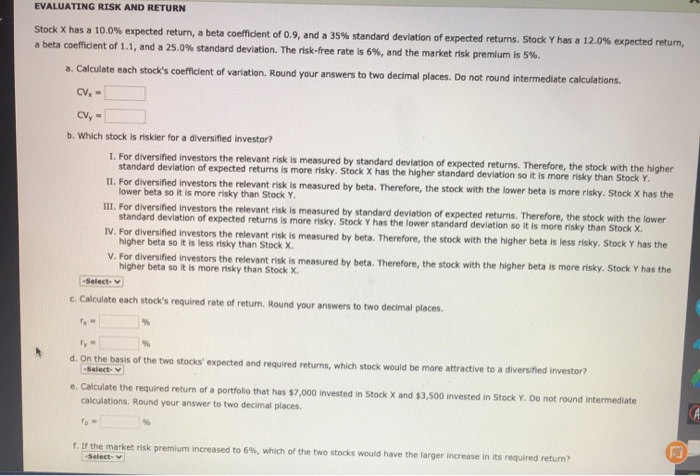EVALUATING RISK AND RETURN Stock X has a 10.0% expected return, a beta coefficient of 0.9, and a 35% standard deviation of expected returns. Stock Y has a 12.0% expected return, a beta coefficient of 1.1, and a 25.0% standard deviation. The risk-free rate is 6%, and the market risk premium is 5%. a. Calculate each stock's coefficient of variation. Round your answers to two decimal places. Do not round intermediate calculations. CV, - CV- b. Which stock is riskler...

• The following graph plots the current security market line (SML) and indicates the return that investors...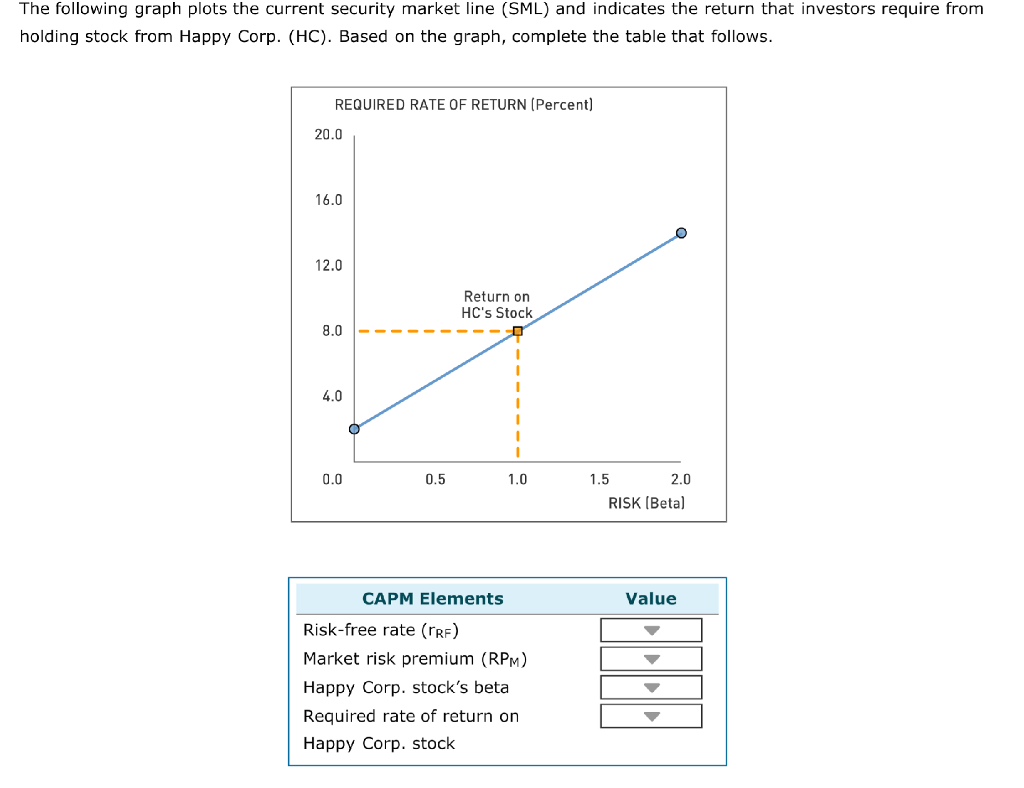The following graph plots the current security market line (SML) and indicates the return that investors require from holding stock from Happy Corp. (HC). Based on the graph, complete the table that follows. REQUIRED RATE OF RETURN (Percent] 20.0 16.0 12.0 Return on HC's Stock 8.0 4.0 0.5 1.0 1.5 2.0 RISK (Betal 0.0 CAPM Elements Value Risk-free rate (RF) Market risk premium (RPM) Happy Corp. stock's beta Required rate of return on Happy Corp. stock An analyst believes that...

• Excel Online Structured Activity: Evaluating risk and return Stock X has a 9.5% expected return, a...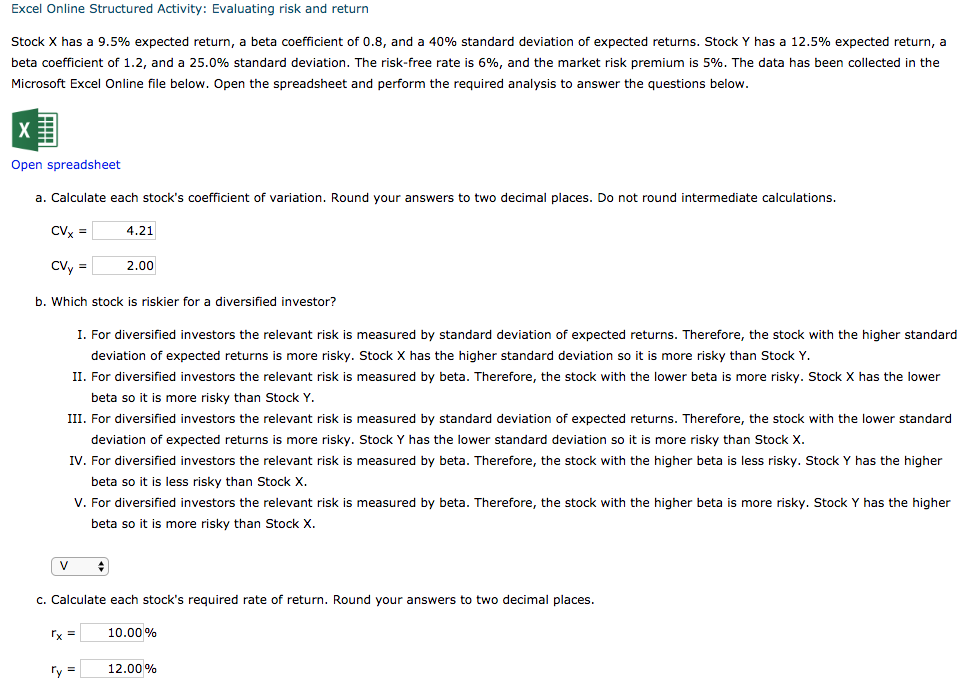Excel Online Structured Activity: Evaluating risk and return Stock X has a 9.5% expected return, a beta coefficient of 0.8, and a 40% standard deviation of expected returns. Stock Y has a 12.5% expected return, a beta coefficient of 1.2, and a 25.0% standard deviation. The risk-free rate is 6%, and the market risk premium is 5%. The data has been collected in the Microsoft Excel Online file below. Open the spreadsheet and perform the required analysis to answer the...